# 4.1 Maximum and Minimum Values

## Fermat's Theorem

Fermat's Theorem: If $$f$$ has local max or min at $$c$$, and if $$f'(c)$$ exists, then $$f'(c)=0.$$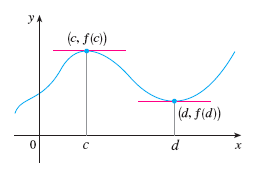The converse is not true. ATTENTION: $$f'(c)=0$$ does not mean $$f(c)$$ is a local max or min.
Counter example. $$f(x)=x^3$$. $$f'(0)=0$$ but $$f$$ has no max or min (local or global).

Example of Fermat's Theorem. $$f(x)=\sin x$$ has a local max at $$x=\frac{\pi}{2}$$ and $$f'(\frac{\pi}{2})=0$$.

## Critical Numbers (or Critical Points)

A critical number of a function is a number in the domain of $$f$$ such that either $$f'(c)=0$$ or $$f'(c)$$ does not exist.

Example 1. Find the critical numbers of $$f(x)=6x^3-9x^2-36x.$$
Sol: Solving $$f'(x)=0$$ yields $$x=-1$$ and $$x=2$$. So $$-1, 2$$ are critical numbers.

Example 2. Find the critical numbers of $$f(x)=\sqrt{x}.$$
Sol: Domain of $$f$$: $$x\geq 0$$. Derivative is $$f'(x)=\frac{1}{2}x^{-1/2}$$. Note that $$f'(x)\neq 0$$ for $$x>0$$ and $$f'(0)$$ does not exist. Hence the only critical number is $$0$$.
(Comment: this is an example where $$0$$ is in the domain of $$f$$ and $$f'(0)$$ does not exist)

## Finding Max & Min using The Closed Interval Method

The Closed Interval Method for finding global max & min of continuous $$f$$ over $$[a,b]$$:
1. Find values of $$f$$ at endpoints of the interval: $$f(a)$$, $$f(b)$$
2. Find values of $$f$$ at all critical points: first find all critical points, then evaluate $$f$$ at those pts
3. Choose the largest from Steps 1,2 as max and the smallest as min

Example. Find the global max and min of $f(x)=x^3-3x^2+1, \quad -\frac{1}{2}\leq x\leq 4$
Sol:
Step 1. Find values of $$f$$ at endpoints:

endpts $$f$$ values
$$\frac{1}{2}$$ $$\frac{1}{8}$$
$$4$$ $$17$$

Step 2. Find all critical pts and values of $$f$$ at those pts. Since $$f'(x)$$ exists for all $$x$$, all critical pts are roots of $f'(x)=3x^2-6x=0.$ Hence the critical numbers are $$x=0$$ and $$x=2$$.
Values of $$f$$ at critical pts are

critical pts $$f$$ values
$$0$$ $$1$$
$$2$$ $$-3$$

Step 3. Candidates of extreme values are $$\frac{1}{8}, 17, 1, -3$$. We see that: max = $$17$$, min = $$-3$$.

# 4.3 Derivatives and the Shape of a Graph

## $$f'$$ and the monotonicity of $$f$$

• If $$f'(x)>0$$ on an interval, then $$f$$ is increasing on that interval
• If $$f'(x)<0$$ on an interval, then $$f$$ is decreasing on that interval

Example. Find where the function $$f(x)=3x^4-4x^3-12x^2+5$$ is increasing or decreasing.
Sol: $f'(x)=12x^3-12x^2-24x=12x(x-2)(x+1).$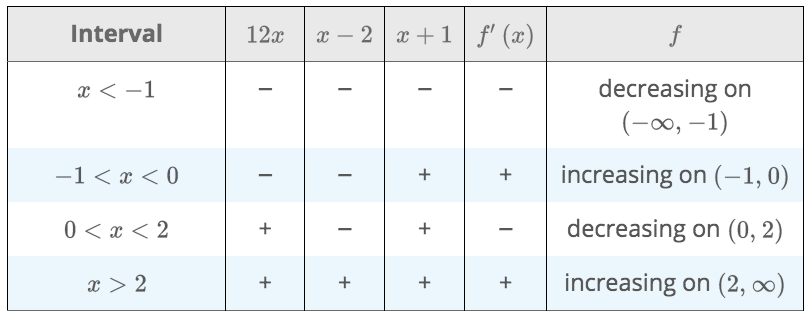## The First Derivative Test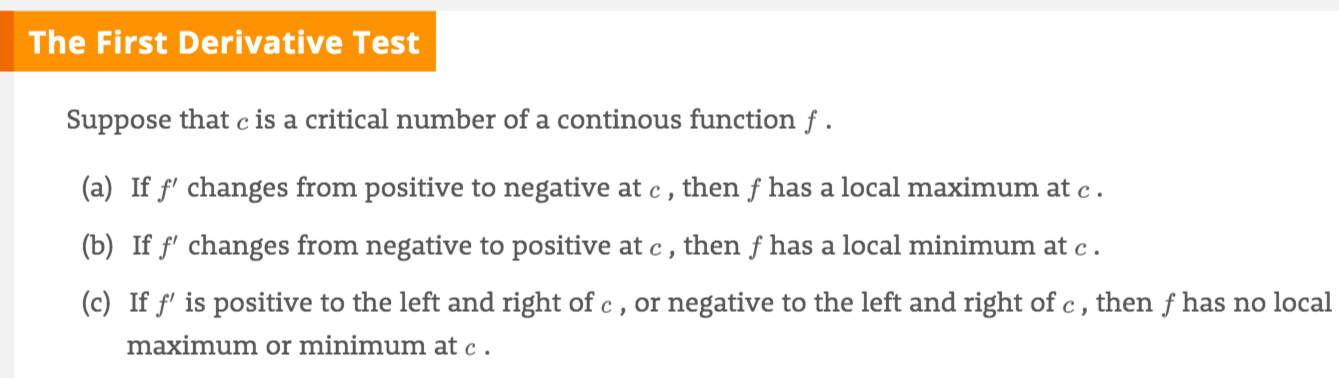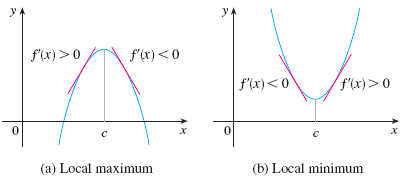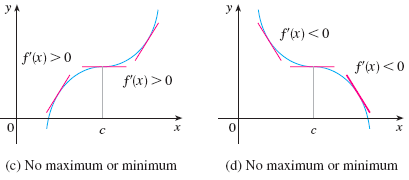Ex. Find the local max and min of $$f(x)=3x^4-4x^3-12x^2+5$$.

## $$f''$$ and the concavity of $$f$$

concave upward (CU) and concave downward (CD)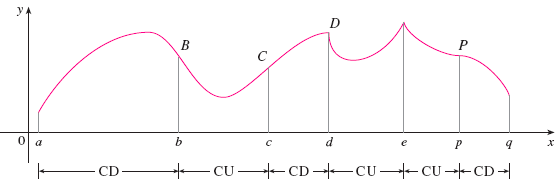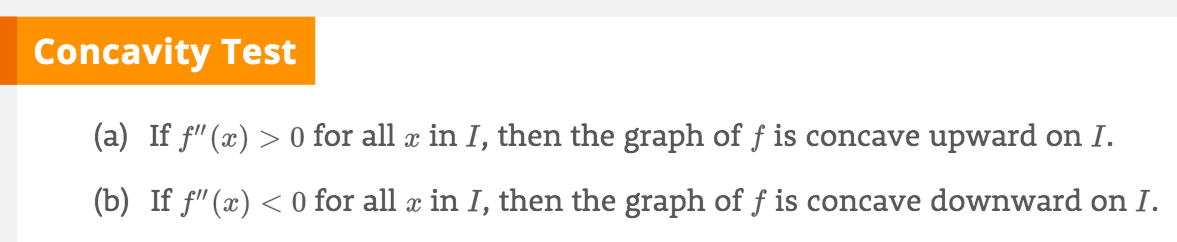If $$f''(c)=0$$, then $$c$$ is called an inflection point

## The Second Derivative Test

• $$f''(c)>0$$ ==> concave upward ==> $$f$$ has a local min at $$c$$
• $$f''(c)<0$$ ==> concave downward ==> $$f$$ has a local max at $$c$$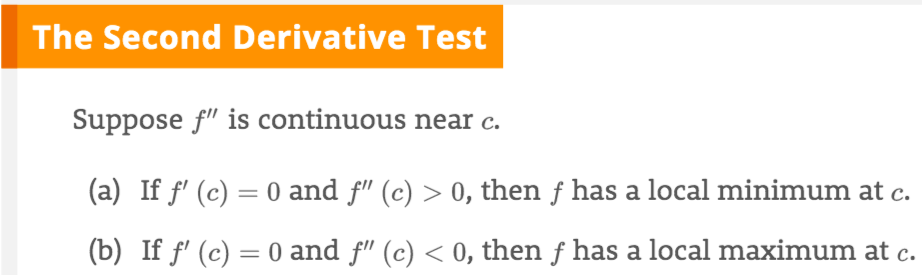Example. $$f''$$ is continuous near $$c$$. $$f'(1)=0$$ and $$f''(1)=-1$$. What can you say about $$f$$ at $$x=1$$ ?
Sol: $$f(1)$$ is a local maximum.

Ex. Discuss the curve $$y=x^3$$ in terms of monotonicity, concavity, inflection point, max/min, etc.